# Martin Orr's Blog

Maths > Abelian varieties > Periods of abelian varieties

## Period relations on abelian varieties

Posted by Martin Orr on Monday, 26 October 2015 at 11:00

The Legendre period relation is a classical equation relating the periods and quasi-periods of an elliptic curve, as defined last time. I will discuss this relation, and then more generally discuss how the existence of polarisations implies relations between the periods of higher-dimensional abelian varieties.

These examples motivate the introduction of the geometric motivic Galois group, which gives an upper bound for the transcendence degree of periods of an abelian variety (or indeed any algebraic variety). This upper bound is conjectured to be equal to the actual transcendence degree. I had intended to discuss the geometric motivic Galois group in this post too, but I decided that it was getting to long so I will postpone that to another time.

### The Legendre period relation

Letbe an elliptic curve over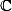with equation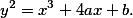Recall that there is a basisforis represented by the differential forms of the second kind(which is regular) and(which has a double pole at infinity). If we choose a basis,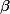for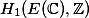, then we can define the fundamental periods ofasand the quasi-periods asThe Legendre period relation asserts that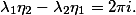In the language of the previous post, the determinant of the extended period matrix ofis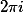. Note that the sign in this equation (or) depends on the ordering ofand- this is chosen based on the standard orientation ofto ensure that we end up with).

Following the introduction to Deligne's paper on absolute Hodge classes, I want to give a simple proof that ifis defined over the field, then the Legendre period relation holds up to multiplication by a scalar in:Note that if what we are really interested in is transcendence properties of the periods and quasi-periods, then an identity which holds up to multiplication by k is as good as an exact identity.

The key point is that the de Rham cohomology classes represented byandare defined over. Hence the extended period matrixexpresses a basis forin terms of a basis for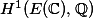, via the standard comparison isomorphismSince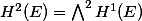, both in de Rham cohomology and in singular cohomology, it follows thatis the coordinate for the basis elementrelative to the basis element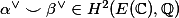.

But there is a pair of bases forandwhich we already know how to compare. We can take the cycle class of a point in each cohomology theory, and we have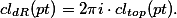Recall that we proved this relation for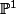, and it motivates the definition of Tate twists of Hodge structures. We can deduce that the same relation holds on an elliptic curve (or indeed any smooth projective curve) by considering a finite morphism, say of degree. The pullback of a point inispoints in, and soand similarly for. Dividing by, the relation (*) forimplies the same relation for.

Sinceand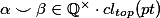, we conclude thatObserve that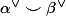is a generator for the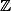-moduleand so in fact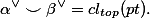Thus showing that the Legendre period relation holds exactly is equivalent to showing thatThis is a purely algebraic result (it no longer involves integration as does the classical statement of the period relation). But I think that a purely algebraic proof of it is hard. Deligne sketches an analytic proof of this algebraic result.

### Algebraic cycles and relations between periods

At first sight it might look like the Legendre period relation provides a lower bound for the transcendence degree of the periods of an elliptic curve - it implies thatunless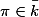. But really one should think of it as providing an upper bound for the transcendence degree over the field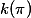: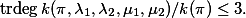Indeed, it does not just tell us that there exists an algebraic relation between the periods and quasi-periods over, it tells us exactly what form that relation takes.

The presence ofin the field generated by periods is inevitable due to the issue of Tate twists. A similar argument to the argument for the Legendre period relation shows that, for any abelian variety of dimensiondefined over, the determinant of the extended period matrix is in.

The Legendre period relation and the more general relation for the determinant of the extended period matrix are examples of the principle that algebraic cycles on a variety imply algebraic relations between the periods. In particular, the determinant relation comes from the fact that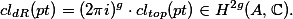For another example of relations between periods implied by an algebraic cycle, consider a polarisation on the abelian variety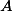. A polarisation is a symplectic pairing onor equivalently an element of. The definition of polarisation requires that it must be of the formfor an ample divisor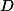on.

The algebraic cyclewill also induce an element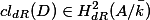and hence a symplectic pairing on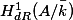. The compatibility of the cycle class maps implies that under the comparison isomorphism, we get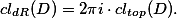Now if we choose symplectic bases forandwith respect to the respective symplectic forms induced by, and use these bases to calculate the extended period matrix, then we will get a matrix in the general symplectic groupThis implies that the transcendence degree overof the extended period matrix must be at most, and for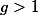this is better than the trivial upper bound of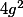.

1. Motivic Galois groups and periods From Martin's Blog

In my last post, I discussed ... an upper bound for the transcendence degree of the extended period matrix of an abelian variety, namely the dimension of the general symplectic group. ... In this post, I will discuss how this can be generalised ...

2. Gross's proof of the Chowla-Selberg formula From Martin's Blog

Today I am going to write about Gross's proof of the Chowla-Selberg formula (up to algebraic numbers). As I discussed last time, the Chowla-Selberg formula is a formula for the periods of a CM elliptic curve E in terms of values of the gamma...